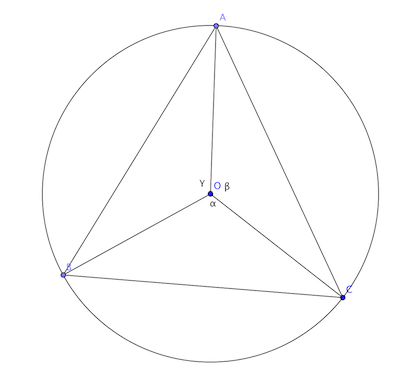Trigonometry! #103

Geometry Level 5The sides of a triangle inscribed in a given circle subtend angles $\alpha$, $\beta$ and $\gamma$ at the centre. Then find the minimum value of the arithmetic mean of $\cos\left(\alpha+\frac{\pi}{2}\right)$, $\cos\left(\beta+\frac{\pi}{2}\right)$ and $\cos\left(\gamma+\frac{\pi}{2}\right)$.

If the minimum value can be expressed as $- \sqrt{ \frac{a}{b} }$, where $a$ and $b$ are coprime positive integers. What is the value of $a + b$?

This problem is part of the set Trigonometry.

×